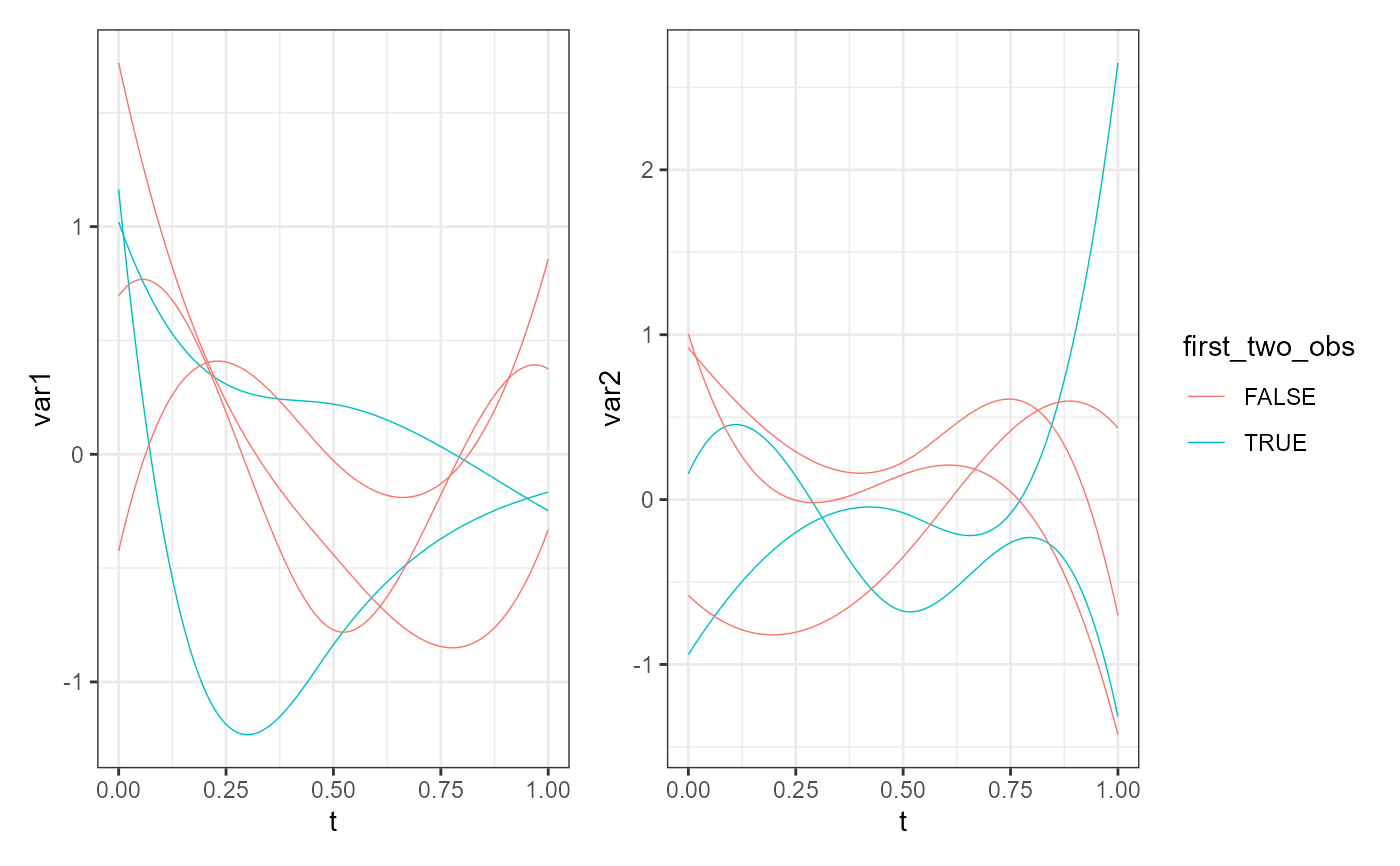Plot an object of class mfd using ggplot2 and patchwork.

plot_mfd(
mfdobj,
mapping = NULL,
data = NULL,
stat = "identity",
position = "identity",
na.rm = TRUE,
orientation = NA,
show.legend = NA,
inherit.aes = TRUE,
type_mfd = "mfd",
y_lim_equal = FALSE,
...
)

## Arguments

mfdobj

A multivariate functional data object of class mfd.

mapping

Set of aesthetic mappings additional to x and y as passed to the function ggplot2::geom:line.

data

A data.frame providing columns to create additional aesthetic mappings. It must contain a factor column "id" with the replication values as in mfdobj$fdnames[]. If it contains a column "var", this must contain the functional variables as in mfdobj$fdnames[].

stat

See ggplot2::geom_line.

position

See ggplot2::geom_line.

na.rm

See ggplot2::geom_line.

orientation

See ggplot2::geom_line.

show.legend

See ggplot2::geom_line.

inherit.aes

See ggplot2::geom_line.

type_mfd

A character value equal to "mfd" or "raw". If "mfd", the smoothed functional data are plotted, if "raw", the original discrete data are plotted.

y_lim_equal

A logical value. If TRUE, the limits of the y-axis are the same for all functional variables. If FALSE, limits are different for each variable. Default value is FALSE.

...

See ggplot2::geom_line.

## Value

A plot of the multivariate functional data object.

## Examples

library(funcharts)
library(ggplot2)
mfdobj <- data_sim_mfd()
ids <- mfdobj\$fdnames[]
df <- data.frame(id = ids, first_two_obs = ids %in% c("rep1", "rep2"))
plot_mfd(mapping = aes(colour = first_two_obs),
data = df,
mfdobj = mfdobj)